# CAT Geometry Questions | CAT Questions

### CAT Verbal Ability

CAT Geometry is an important topic that is always covered in MBA exams. It is important because MBA aspirants need to have the diagnostic skill to understand shapes and figures. Some of the topics that are being covered as geometry basics are circles, triangles, parallel lines. Congruency and Similarity of triangles is a chapter that is being intensively concentrated on geometry. A candidate can easily expect 2-3 Geometry question in CAT and also in other MBA exams such as NMAT, XAT, SNAP, etc.

## CAT Quantitative Aptitude questions | CAT Geometry

1.  A circle is inscribed in a rhombus with diagonals 12 cm and 16 cm. The ratio of the area of circle to the area of rhombus is

[CAT 2020]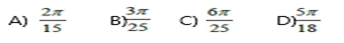Area of rhombus = 12*16/2 = 96

Let h = radius ; a = diagonal1; b = diagonal2

a = 12

b = 16

AB^2 = (a/2)^2 + (b/2)^2 = 6^2+8^2 = 36+64 = 100

AB = 10

(a/2 * b/2) / 2 = AB * h/2

6*8 = 10*h

H = 4.8

Area of circle = Pi * 4.8 * 4.8

Ratio of areas = Pi * 4.8 * 4.8 /96

On solving = Pi * 6/25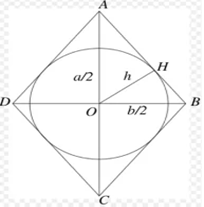2.  In a circle of radius 11 cm, CD is a diameter and AB is a chord of length 20.5 cm. If AB and CD intersect at a point E inside the circle and CE has length 7 cm, then the difference of the lengths of BE and AE, in cm, is

A) 2.5    B) 3.5    C) 0.5    D) 1.5                                                                                                                        [CAT 2019]

As per question following figure can be drawn,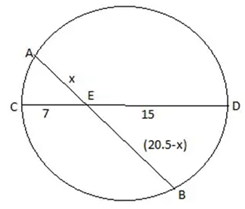As we know AE*BE = CE*DE

X(20.5 –x) = 7*15 = 105

20.5x –x^2 = 105

x^2 -20.5x + 105 = 0

x^2 -10.5x – 10x +105 =0

(x -10)*(x-10.5) =0

So x = 10 or 10.5

Thus if AE = 10 then BE = 10.5 and vice versa

Required difference = (10.5 -10) = 0.5

3.  Two circles, each of radius 4 cm, touch externally. Each of these two circles is touched externally by a third circle. If these three circles have a common tangent, then the radius of the third circle, in cm, is

A)  π/3     B) 1/√2     C) √2     D) 1                                                                                                                          [CAT 2019]

See the figure,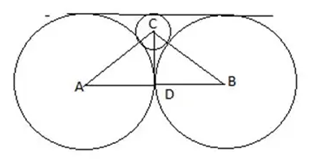In above figure, AD=BD =4, let radius of 3rd circle = r
So AC = 4+r, CD = 4-r
Using Pythagoras theorem in right angled triangle ADC,
(4+r) ^2 = 4^2 + (4-r)^2
16+ r^2 + 8r = 16 + 16 + r^2 – 8r

4.  In a circle, two parallel chords on the same side of a diameter have lengths 4 cm and 6 cm. If the distance between these chords is 1 cm, then the radius of the circle, in cm, is

A)  12     B) √14     C) √13     D) √11                                                                                                                      [CAT 2018]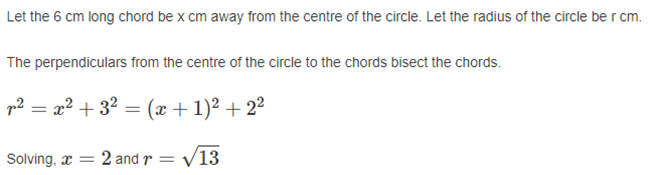## Basic Concepts in CAT Geometry:

The list of concepts that are covered in basic CAT Geometry are as follows: –

• Basic Properties of Triangle Geometry: – Some basic properties are given below: –
1. The sum of the three interior angles is 180 degrees.
2. The measure of an exterior angle is equal to the sum of the measures of two remote interior angles.
3. The sum of the three exterior angles is 360 degrees.
4. The side opposite to the greatest angle is the longest, and the side opposite to the smallest angle is the shortest
• Types/Sides of Triangles: For eg: – Consider an obtuse triangle with sides 8cm, 15cm & x cm. How many such triangles are possible?
• Congruency/Similarity of triangles: – For eg: – How many noncongruent triangles are possible such that their sides are integers, and the perimeter is equal to 14.
• Some basic geometry concepts like Important theorems / formula / methods in geometry to study: –
1. Angle Bisector Theorem
2. Pythagoras Theorem
3. Midpoint Theorem
4. Basic Proportionality Theorem
5. Heron’s Formula
6. Trigonometric Formulas
7. Determinant Method
• Some Important Lines and Points to Study in geometry are: –
1. Altitude
2. Orthocentre
3. Perpendicular Bisector
4. Circumcentre
5. Median
6. Centroid
7. Angle Bisector
8. Incentre
• Find the angle / sum of angles of the shape inscribed in another shape.
• Find the length of a side/sides, area of the shape, or shape inscribed within another shape.

## How to deal with the Topic Preparation:

### Level 1

• Students can learn the basic concept in CAT Geometry and properties of different shapes / lines-triangles, circle, rectangles, polygons, parallel lines, perpendicular lines, etc by using MBAP CAT E-book (Concept Theory) study material.
• Students should develop diagnostic skills to draw and find solutions by referring to MBAP Live Lecture Recordings (Basic) on the basic concepts.
• Students need to have the skill to identify which concept / method / formula / theorem to use. These skills can be gained by the students by practicing from MBAP CAT E-book (Practice questions) and also by taking up MBAP lecture Assignment.

### Level 2

• Topics such as congruency / similarity of triangles, shape inscribed inside another shape, theorems in geometry are focused intensively. So, students need to prepare well and practice problems on geometry.
• The geometry section in the QA is one of the easiest sections to attempt if the students have done their part properly. It is recommended that students should use MBAP Topic Wise Previous Year CAT Questions to practice and ace this section in the exam.

### Level 3

• Students need to look into problems that use multiple basic concept of geometry to find a solution. Try to answer advanced level of geometry CAT questions from previous papers. You can practice the advanced level questions by using MBAP CAT Advance E-books.  And try to attempt these questions in a time-based manner. Taking too much time for advanced questions in geometry section will be disadvantageous in cracking the exam. Finally, students need to practice as many questions as they can to get a hold of the concepts and find solutions fast. Another best practice is to solve questions from MBAP Previous year CAT Paper. Practice will always help the student to crack the exam.Counselling Session
By IIM Mentor

#### Words From Our StudentsNihar Mehta
Calls from IIMs, SPJIMR & MDI
During my CAT preparation, I enrolled at MBA Pathshala for classroom coaching. Abhijit Sir & Haider Sir guided me through the process of planning & executing the strategies I had to use to succeed in the test. I received regular feedback on my progress during the GD and PI preparations after CAT. I received calls from IIMs, SPJIMR, and MDI. MBAP played a huge role in this, and I cannot thank them enough for their efforts.Ishan Rathod
Calls from IIM I, S, and all New And Baby IIM's.
MBAP is a great place to prepare for MBA-CET, CAT, and CMAT, and the faculty are unbeatable. Most importantly, they pay personal attention to each student. Most faculty members come from IIMs. One-on-one interaction helped me improve as the exams approached, as well as get calls from IIMs I, S, and all New and Baby IIMs. Interview sessions were very beneficial for me to convert IIM S.Sanskriti Reja
Calls from IIMs, XLRI, XIMB
I am glad I chose MBA Pathshala for my MBA coaching. The faculty here is excellent and very competent. If I had any questions or needed extra classes, they always gave me one. I got through my GD-PIs too after the exams with their help. Thanks to MBAP, I was able to pass CAT and other entrance exams. Among others, I received calls from XLRI, XIMB, and IIMs. A few of the colleges I've converted are KJ Somaiya, TAPMI, IIM Sambalpur, and IIM Sirmaur.Parth Kanakia
Converted SPJIMR
MBAP prepared me for GD, WAT, and PI. Abhijit Singh, Haider, and other MBAP faculty provided excellent training. Good knowledge was imparted from the very beginning. Regular mock interviews were conducted. As a former SPJIMR, I would recommend students to join MBAP.Ronit jaiswal
Calls from IIM-A/B/C & SNAP 98.12%ile
Coaching offers me personalized attention and a competitive environment. Because the preparation was so rigorous, I eventually began to perform at my best. The mock interviews/GD/WATs were conducted by anonymous panels, and it really boosted my confidence. Although I got a call from SCMHRD (SNAP-98.12), Abhijeet sir felt that I should try again and aim for IIM-A/B/C. It has been your pleasure to have been your mentor.Maitreya Khanapurkar
Calls From IIMs
You will get good support from the faculty until you get into a B-School. The shortcut techniques you will learn will be extremely useful. You will be able to resolve doubts at any time. If you plan on taking CAT CET or any other entrance exam, go for MBA Pathshala. With the help of MBAP tutors, I received a call from IMT Ghaziabad.
Yash Dandavate
GMAT Score - 660
GMAT prep with MBAP was the best I've ever experienced. With Rohan Kulkarni Sir's Verbal Guidance, I scored 85th percentile in my GMAT exam as I was very weak in English. I always found Haider Sir and Abhijeet to answer my questions and to develop strategies and shortcuts to tackle GMAT problems for me. MBAP is a great option for GMAT and CAT prep.

#### Free Material Area